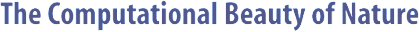Computer Explorations of Fractals, Chaos,Complex Systems, and Adaptation· title page · home* · cover artwork · jacket text · table of contents · the author* · ordering informationBook Contents
 · three themes · part synopses · selected excerpts · all figures from book · quotes from book · glossary from book · bibliography · slide showSource Code
 · overview &documentation · FAQ list* · download source code · java appletsMiscellany
 · news* · reviews & awards · errata · for educators · bibliography (BibTeX format) · other linksGlossary - M

 [ A | B | C | D | E | F | G | H | I | J | K | L | M | N | O | P | Q | R | S | T | U | V | W | X | Y | Z ]

# M

Mackey-Glass System     A delay differential equation (dx/dt = (ax(t-tau))/(1 + x^10(t-tau)) - bx(t)) that can display a wide variety of behaviors via an adjustable delay term, tau. Even though this system generates a single scalar time series, it can be extremely chaotic because its value at any time may depend on its entire previous history.

Mandelbrot Set     An extremely complex fractal that is related to Julia sets in the way that it is constructed and by the fact that it acts as a sort of index to the Julia sets. Like the Julia sets, the Mandelbrot set is calculated via an iterative procedure. Starting conditions that do not diverge after an infinite number of iterations are considered to be inside the set. If, and only if, a complex number is in the Mandelbrot set, then the Julia set that uses that complex number as a constant will be connected; otherwise, the corresponding Julia set will be unconnected.

Map     A function that is usually understood to be iterated in discrete time steps.

Matrix     A rectangular two-dimensional array of numbers that can be thought of as a linear operator on vectors. Matrix-vector multiplication can be used to describe geometric transformations such as scaling, rotation, reflection, and translation. They can also describe the affine transformation used to construct IFS and MRCM fractals.

Mean     The arithmetical average of a collection of numbers; the center of a Gaussian distribution.

Meme     A unit of cultural information that represents a basic idea that can be transferred from one individual to another, and subjected to mutation, crossover, and adaptation.

Message     The basic unit of information in a classifier system that is stored in the message list. A message may correspond to an external state of an environment or an internal state of the classifier system.

Message List     The portion of a classifier system that retains information in the form of messages.

Mixed Strategy     In game theory, a strategy that uses randomness by employing different actions in identical circumstances with different probabilities.

Model     In the sciences, a model is an estimate of how something works. A model will usually have inputs and outputs that correspond to its real-world counterpart. An adaptive system also contains an implicit model of its environment that allows it to change its behavior in anticipation of what will happen in the environment.

Model of Computation     An idealized version of a computing device that usually has some simplifications such as infinite memory. A Turing machine, the lambda calculus, and Post production systems are all models of computation.

Monotonic     The property of a function that is always strictly increasing or strictly decreasing, but never both. The sigmoidal activation function of a multilayer perceptron is monotonically increasing.

MRCM     The Multiple Reduction Copy Machine algorithm, which can be used to make affine fractals. MRCM fractals are related to IFS fractals in that they both use the same types of affine transformations. The MRCM algorithm performs several affine transformations of a seed image in parallel to yield a secondary seed image. The output of the MRCM is recursively passed back through to its input multiple times, to yield the fractal.

Multilayer Perceptron (MLP)     A type of feedforward neural network that is an extension of the perceptron in that it has at least one hidden layer of neurons. Layers are updated by starting at the inputs and ending with the outputs. Each neuron computes a weighted sum of the incoming signals, to yield a net input, and passes this value through its sigmoidal activation function to yield the neuron's activation value. Unlike the perceptron, an MLP can solve linearly inseparable problems.

Mutation     A random change in any portion of genetic material. For a genetic algorithm, this means that a value in a bit string is randomly set.

 [ A | B | C | D | E | F | G | H | I | J | K | L | M | N | O | P | Q | R | S | T | U | V | W | X | Y | Z ]Copyright © Gary William Flake, 1998-2002. All Rights Reserved.Last modified: 30 Nov 2002